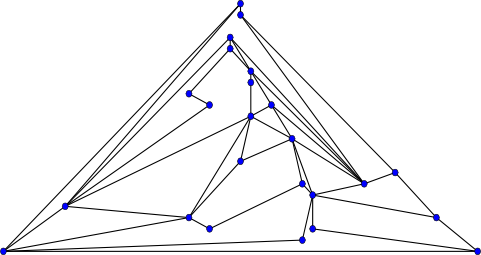#Boost C++ Libraries

...one of the most highly regarded and expertly designed C++ library projects in the world.

This is the documentation for an old version of Boost. Click here to view this page for the latest version.# Chrobak-Payne Straight Line Drawing

```template<typename Graph,
typename PlanarEmbedding,
typename ForwardIterator,
typename PositionMap,
typename VertexIndexMap>
void chrobak_payne_straight_line_drawing(const Graph& g,
PlanarEmbedding perm,
ForwardIterator ordering_begin,
ForwardIterator ordering_end,
PositionMap drawing,
VertexIndexMap vm
);
```

A straight line drawing of a planar graph is a plane drawing where each edge is drawn using a straight line segment. Since all edges are line segments, the drawing is completely determined by the placement of vertices in the plane. chrobak_payne_straight_line_drawing uses an algorithm of Chrobak and Payne  to form a straight line drawing of a planar graph by mapping all n vertices in a planar graph to integer coordinates in a (2n - 4) x (n - 2) grid.The input graph passed to chrobak_payne_straight_line_drawing must be a maximal planar graph with at least 3 vertices. Self-loops and parallel edges are ignored by this function. Note that the restriction that the graph be maximal planar does not mean that this function can only draw maximal planar graphs (the graph pictured above is not maximal planar, for example). If you want to draw a graph g, you can create a copy g' of g, store a mapping m of vertices in g' to vertices in g, triangulate g', and then send g' in as the input to chrobak_payne_straight_line_drawing. The drawing returned can then be applied to g using m to translate vertices from one graph to another, since g contains a subset of the edges in g'.

### Complexity

If the vertex index map provides constant-time access to indices, this function takes time O(n + m) for a planar graph with n vertices and m edges. Note that in a simple planar graph with f faces, m edges, and n vertices, both f and m are O(n).

### Parameters

IN: Graph& g
An undirected graph. The graph type must be a model of Vertex List Graph
IN PlanarEmbedding embedding
A Readable Property Map that models the PlanarEmbedding concept.
IN ForwardIterator
A ForwardIterator that has value_type equal to graph_traits<Graph>::vertex_descriptor.
OUT: PositionMap
A Writable LValue Property Map that models the Position Map concept. The Position Map concept requires that the value mapped to be an object that has members x and y. For example, if p models PositionMap and v is a vertex in the graph, p[v].x and p[v].y are valid expressions. The type of x and y must be implicitly convertable to std::size_t.
IN: VertexIndexMap vm
A Readable Property Map that maps vertices from g to distinct integers in the range [0, num_vertices(g) )
Default: get(vertex_index,g)

### See Also

Copyright © 2007 Aaron Windsor ( aaron.windsor@gmail.com)